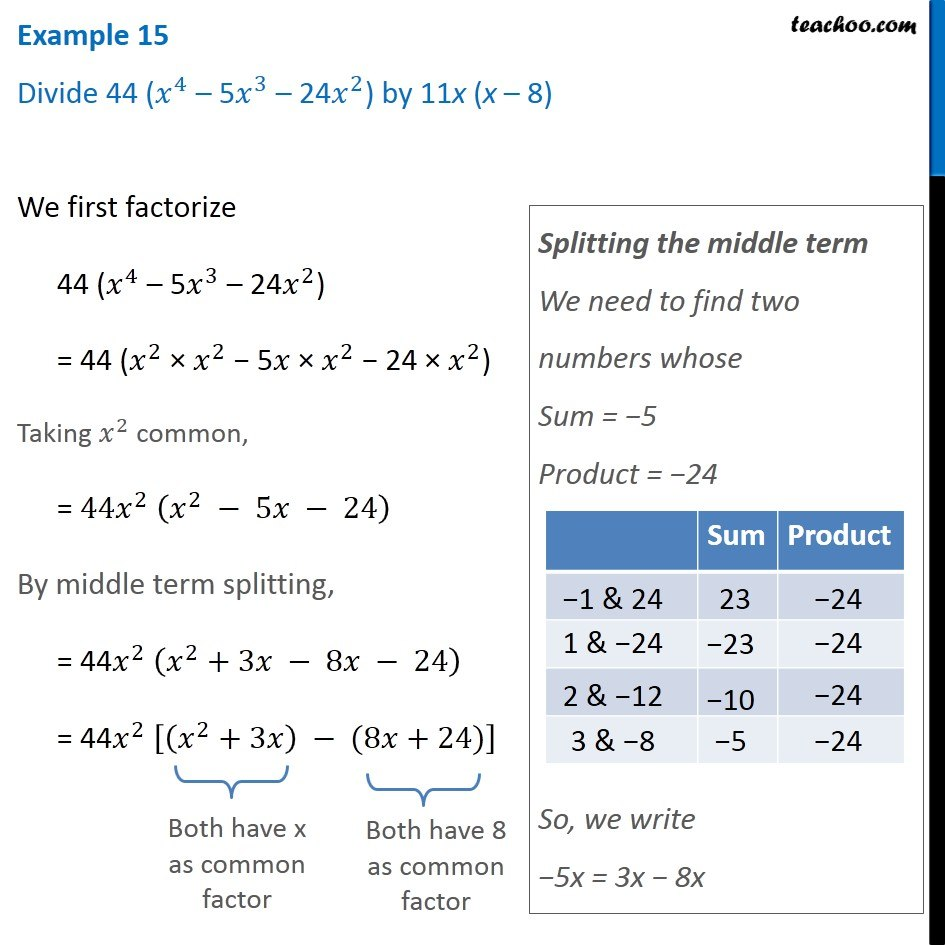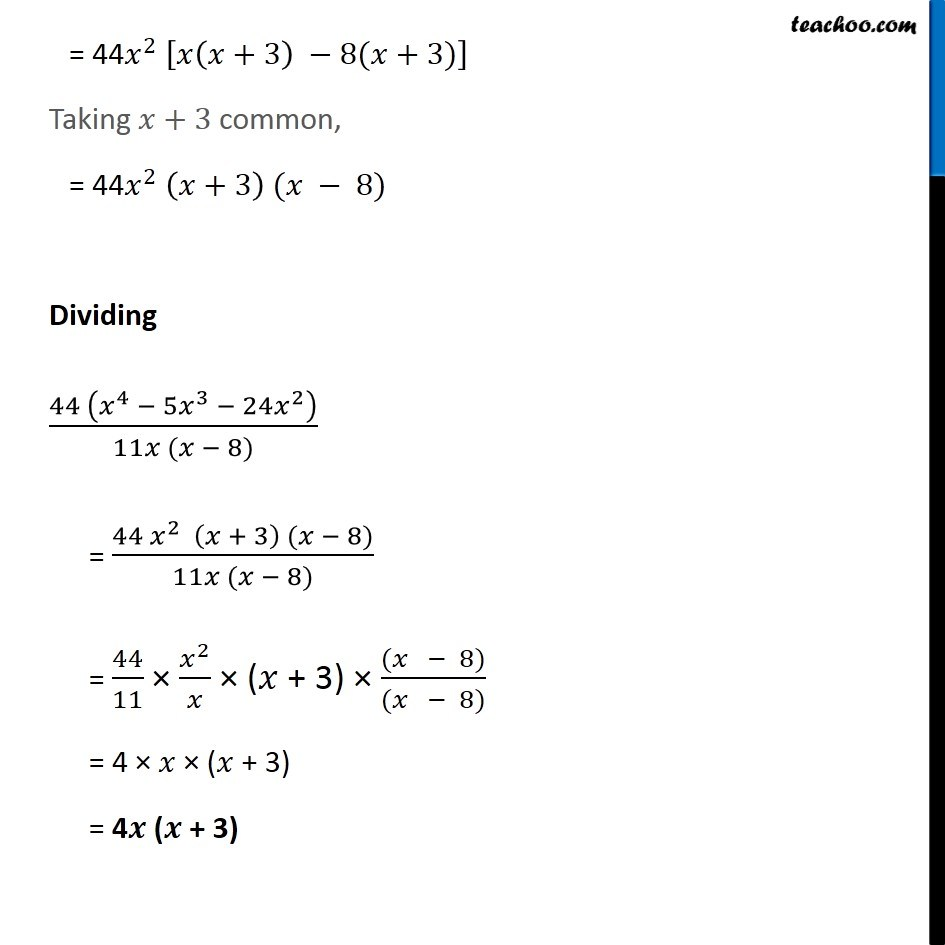Dividing two polynomials

Chapter 14 Class 8 Factorisation
Concept wiseIntroducing your new favourite teacher - Teachoo Black, at only ₹83 per month

### Transcript

Example 15 Divide 44 (𝑥^4 – 5𝑥^3 – 24𝑥^2) by 11x (x – 8) We first factorize 44 (𝑥^4 – 5𝑥^3 – 24𝑥^2) = 44 (𝑥^2 × 𝑥^2 − 5𝑥 × 𝑥^2 − 24 × 𝑥^2) Taking 𝑥^2 common, = 〖44𝑥〗^2 (𝑥^2 − 5𝑥 − 24) By middle term splitting, = 44𝑥^2 (𝑥^2+3𝑥 − 8𝑥 − 24) = 44𝑥^2 [(𝑥^2+3𝑥) − (8𝑥+24)] Both have x as common factor Both have 8 as common factor Splitting the middle term We need to find two numbers whose Sum = −5 Product = −24 So, we write −5x = 3x − 8x = 44𝑥^2 [𝑥(𝑥+3) −8(𝑥+3)] Taking 𝑥+3 common, = 44𝑥^2 (𝑥+3) (𝑥 − 8) Dividing (44 (𝑥^4 − 5𝑥^3 − 24𝑥^2 ))/(11𝑥 (𝑥 − 8)) = (44 𝑥^2 (𝑥 + 3) (𝑥 − 8))/(11𝑥 (𝑥 − 8)) = 44/11 × 𝑥^2/𝑥 × (𝑥 + 3) × ((𝑥 − 8))/((𝑥 − 8)) = 4 × 𝑥 × (𝑥 + 3) = 4𝒙 (𝒙 + 3)